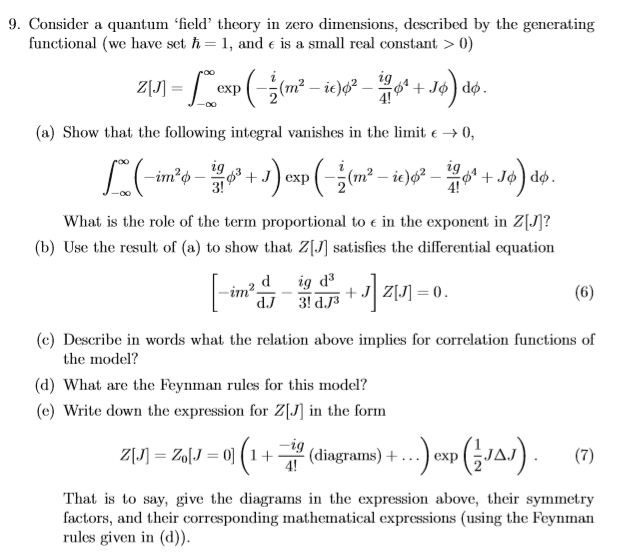# Quantum field theory and generating functional

## Homework Statement

Hi,

I am looking at the attached question, parts a) and b).## The Attempt at a Solution

so for part a) it vanishes because in the ##lim \epsilon \to 0 ## we have a complete derivative:
## \int d\phi \frac{d}{d\phi} (Z[J]) ##

for part b) we attain part a) which we have just shown vanishes by taking the derivatives inside the integral, however, for this to be a complete derivative again we also need that ##lim \epsilon \to 0 ## don't we? but part b) imposes no condition on ##\epsilon## so isn't it asking us to show that this holds for any ##\epsilon## how would we do this instead?

bump
many thanks

nrqed
Homework Helper
Gold Member

## Homework Statement

for part b) we attain part a) which we have just shown vanishes by taking the derivatives inside the integral, however, for this to be a complete derivative again we also need that ##lim \epsilon \to 0 ## don't we? but part b) imposes no condition on ##\epsilon## so isn't it asking us to show that this holds for any ##\epsilon## how would we do this instead?

I agree that they are not being very clear. But I am sure it i implicit that epsilon is taken to zero. The epsilon is only needed to make the integral convergent so if one actually wants to integrate it, the epsilon must be kept and then set to zero after the integration is carried out. But in all other relations like the one given in b), it is understood that the epsilon is taken to be zero. To summarize, the epsilon is alway understood to be zero except when one is actually carrying out integrations.